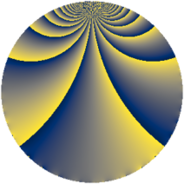# Properties

 Label 784.2.aLevel $784$ Weight $2$ Character orbit 784.a Rep. character $\chi_{784}(1,\cdot)$ Character field $\Q$ Dimension $18$ Newform subspaces $14$ Sturm bound $224$ Trace bound $11$

# Related objects

## Defining parameters

 Level: $$N$$ $$=$$ $$784 = 2^{4} \cdot 7^{2}$$ Weight: $$k$$ $$=$$ $$2$$ Character orbit: $$[\chi]$$ $$=$$ 784.a (trivial) Character field: $$\Q$$ Newform subspaces: $$14$$ Sturm bound: $$224$$ Trace bound: $$11$$ Distinguishing $$T_p$$: $$3$$, $$5$$, $$11$$

## Dimensions

The following table gives the dimensions of various subspaces of $$M_{2}(\Gamma_0(784))$$.

Total New Old
Modular forms 136 23 113
Cusp forms 89 18 71
Eisenstein series 47 5 42

The following table gives the dimensions of the cuspidal new subspaces with specified eigenvalues for the Atkin-Lehner operators and the Fricke involution.

$$2$$$$7$$FrickeDim.
$$+$$$$+$$$$+$$$$4$$
$$+$$$$-$$$$-$$$$6$$
$$-$$$$+$$$$-$$$$5$$
$$-$$$$-$$$$+$$$$3$$
Plus space$$+$$$$7$$
Minus space$$-$$$$11$$

## Trace form

 $$18q + 2q^{5} + 16q^{9} + O(q^{10})$$ $$18q + 2q^{5} + 16q^{9} - 6q^{11} + 2q^{13} - 2q^{15} + 2q^{17} + 8q^{19} + 6q^{23} + 4q^{25} - 4q^{29} + 8q^{31} + 10q^{37} + 20q^{39} - 6q^{41} + 16q^{43} + 10q^{45} - 24q^{47} + 14q^{51} + 2q^{53} - 8q^{55} - 6q^{57} - 6q^{61} - 12q^{65} - 30q^{67} - 16q^{69} + 8q^{71} + 2q^{73} + 32q^{75} + 6q^{79} + 2q^{81} + 8q^{83} - 10q^{85} + 16q^{87} + 2q^{89} - 42q^{93} + 66q^{95} + 18q^{97} + 20q^{99} + O(q^{100})$$

## Decomposition of $$S_{2}^{\mathrm{new}}(\Gamma_0(784))$$ into newform subspaces

Label Dim. $$A$$ Field CM Traces A-L signs $q$-expansion
$$a_2$$ $$a_3$$ $$a_5$$ $$a_7$$ 2 7
784.2.a.a $$1$$ $$6.260$$ $$\Q$$ None $$0$$ $$-3$$ $$-1$$ $$0$$ $$+$$ $$+$$ $$q-3q^{3}-q^{5}+6q^{9}+q^{11}+2q^{13}+\cdots$$
784.2.a.b $$1$$ $$6.260$$ $$\Q$$ None $$0$$ $$-2$$ $$0$$ $$0$$ $$-$$ $$-$$ $$q-2q^{3}+q^{9}+4q^{13}-6q^{17}+2q^{19}+\cdots$$
784.2.a.c $$1$$ $$6.260$$ $$\Q$$ None $$0$$ $$-1$$ $$1$$ $$0$$ $$+$$ $$-$$ $$q-q^{3}+q^{5}-2q^{9}-3q^{11}+6q^{13}+\cdots$$
784.2.a.d $$1$$ $$6.260$$ $$\Q$$ None $$0$$ $$-1$$ $$3$$ $$0$$ $$-$$ $$+$$ $$q-q^{3}+3q^{5}-2q^{9}+3q^{11}+2q^{13}+\cdots$$
784.2.a.e $$1$$ $$6.260$$ $$\Q$$ None $$0$$ $$0$$ $$-2$$ $$0$$ $$+$$ $$-$$ $$q-2q^{5}-3q^{9}+4q^{11}-2q^{13}+6q^{17}+\cdots$$
784.2.a.f $$1$$ $$6.260$$ $$\Q$$ $$\Q(\sqrt{-7})$$ $$0$$ $$0$$ $$0$$ $$0$$ $$-$$ $$-$$ $$q-3q^{9}-4q^{11}-8q^{23}-5q^{25}+2q^{29}+\cdots$$
784.2.a.g $$1$$ $$6.260$$ $$\Q$$ None $$0$$ $$1$$ $$-3$$ $$0$$ $$-$$ $$-$$ $$q+q^{3}-3q^{5}-2q^{9}+3q^{11}-2q^{13}+\cdots$$
784.2.a.h $$1$$ $$6.260$$ $$\Q$$ None $$0$$ $$1$$ $$-1$$ $$0$$ $$+$$ $$+$$ $$q+q^{3}-q^{5}-2q^{9}-3q^{11}-6q^{13}+\cdots$$
784.2.a.i $$1$$ $$6.260$$ $$\Q$$ None $$0$$ $$2$$ $$4$$ $$0$$ $$+$$ $$-$$ $$q+2q^{3}+4q^{5}+q^{9}+8q^{15}+2q^{17}+\cdots$$
784.2.a.j $$1$$ $$6.260$$ $$\Q$$ None $$0$$ $$3$$ $$1$$ $$0$$ $$+$$ $$-$$ $$q+3q^{3}+q^{5}+6q^{9}+q^{11}-2q^{13}+\cdots$$
784.2.a.k $$2$$ $$6.260$$ $$\Q(\sqrt{2})$$ None $$0$$ $$0$$ $$0$$ $$0$$ $$+$$ $$+$$ $$q+\beta q^{3}-2\beta q^{5}-q^{9}-6q^{11}+4\beta q^{13}+\cdots$$
784.2.a.l $$2$$ $$6.260$$ $$\Q(\sqrt{2})$$ None $$0$$ $$0$$ $$0$$ $$0$$ $$-$$ $$+$$ $$q+\beta q^{3}+2\beta q^{5}-q^{9}+2q^{11}+4q^{15}+\cdots$$
784.2.a.m $$2$$ $$6.260$$ $$\Q(\sqrt{2})$$ None $$0$$ $$0$$ $$0$$ $$0$$ $$-$$ $$+$$ $$q+2\beta q^{3}+\beta q^{5}+5q^{9}-4q^{11}+3\beta q^{13}+\cdots$$
784.2.a.n $$2$$ $$6.260$$ $$\Q(\sqrt{2})$$ None $$0$$ $$0$$ $$0$$ $$0$$ $$+$$ $$-$$ $$q+\beta q^{3}-\beta q^{5}+5q^{9}+4q^{11}+\beta q^{13}+\cdots$$

## Decomposition of $$S_{2}^{\mathrm{old}}(\Gamma_0(784))$$ into lower level spaces

$$S_{2}^{\mathrm{old}}(\Gamma_0(784)) \cong$$ $$S_{2}^{\mathrm{new}}(\Gamma_0(14))$$$$^{\oplus 8}$$$$\oplus$$$$S_{2}^{\mathrm{new}}(\Gamma_0(49))$$$$^{\oplus 5}$$$$\oplus$$$$S_{2}^{\mathrm{new}}(\Gamma_0(56))$$$$^{\oplus 4}$$$$\oplus$$$$S_{2}^{\mathrm{new}}(\Gamma_0(98))$$$$^{\oplus 4}$$$$\oplus$$$$S_{2}^{\mathrm{new}}(\Gamma_0(112))$$$$^{\oplus 2}$$$$\oplus$$$$S_{2}^{\mathrm{new}}(\Gamma_0(196))$$$$^{\oplus 3}$$$$\oplus$$$$S_{2}^{\mathrm{new}}(\Gamma_0(392))$$$$^{\oplus 2}$$